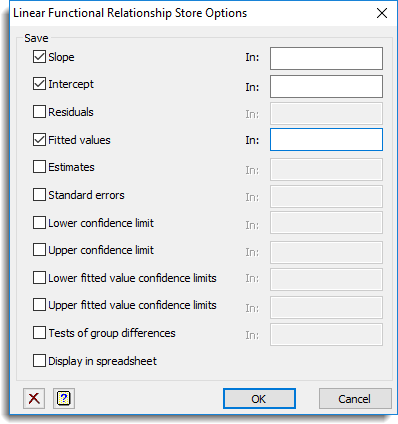1. Home
2. Functional Linear Relationship Store Options

# Functional Linear Relationship Store Options

This dialog lets you save results from a Functional Linear Relationship. These results are saved when an analysis is run and must be specified before running an analysis.

1. After selecting the appropriate boxes, type names for the data structures into the corresponding In: fields.## Save

The table below indicates the type of structures formed for each item.

 Slope Scalar, variate or matrix The slope for each model selected. If only model is selected, this will be a scalar. If groups are defined, then this is a matrix with a column for each group. Intercept Scalar, variate or matrix The intercept for each model selected. If only model is selected, this will be a scalar. If groups are defined, then this is a matrix with a column for each group. Residuals Variate or pointer The residuals from the fitted model will be saved in a variate of this name. If multiple models are selected, then this is a pointer to a set of variates, one for each model. Fitted values Variate or pointer The fitted from the fitted model will be saved in a variate of this name. If multiple models are selected, then this is a pointer to a set of variates, one for each model. Estimates Variate matrix or pointer The estimates for the intercept and slope will be saved in a variate, unless groups are set, when a matrix with a column for each group will be saved. If there are multiple models selected, this will be a pointer to the variates or matrices as described for a single model. Standard Errors of estimates Variate matrix or pointer The standard errors of the estimates for the intercepts and slopes in a variate, unless groups are set, when a matrix with a column for each group will be saved. If there are multiple models selected, this will be a pointer to the variates or matrices as described for a single model. Lower confidence limit Variate matrix or pointer The lower confidence limit of the estimates for the intercepts and slopes in a variate, unless groups are set, when a matrix with a column for each group will be saved. If there are multiple models selected, this will be a pointer to the variates or matrices as described for a single model. Upper confidence limit Variate matrix or pointer The upper confidence limit of the estimates for the intercepts and slopes in a variate, unless groups are set, when a matrix with a column for each group will be saved. If there are multiple variates, this will be a pointer to the variates or matrices as described for a single model. Lower fitted value confidence limit Variate or pointer The lower confidence limit of the fitted values in a variate. If there are multiple models selected, this will be a pointer to a variates for each model. The bootstrapping must be used to obtain these estimates. Upper fitted value confidence limit Variate or pointer The upper confidence limit of the fitted values in a variate. If there are multiple models selected, this will be a pointer to a variates for each model. The bootstrapping must be used to obtain these estimates. Test of group differences Pointer to symmetric matrices The pointer has three elements (labelled ‘slopes’, ‘elevations’ and ‘locations’) which save symmetric matrices. The element on the diagonal of each symmetric matrix contains the overall probability that all groups have the same estimates of slopes, elevations (intercepts assuming the slopes for the groups are the same) and locations (assuming slopes and intercepts for groups are the same), and the lower triangle contains the pairwise probabilities that two groups have the same estimates.Clear Clear all fields and list boxes.Help Open the Help topic for this dialog.